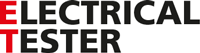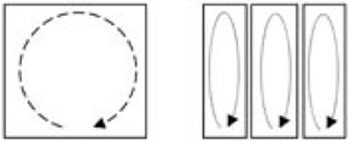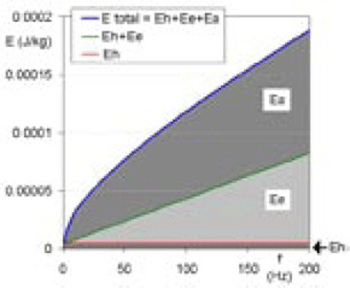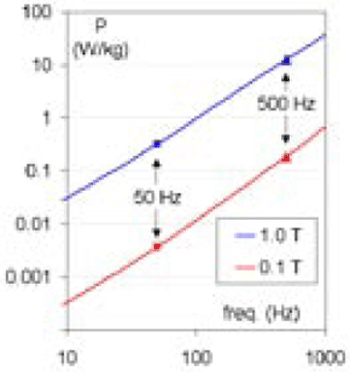ET online
October 2009
Magnetic losses and efficiency in magnetic materials# Magnetic losses and efficiency in magnetic materials

30 October 2009

Dr Stan Zurek - Magnetics technical specialist

Where it all started...

Before we delve deep into our topic let us take a step back in time. As is well known, in the 19th century great scientists like Faraday and Maxwell developed the electromagnetic theory. Around the same time, the first electric motors and transformers were invented, but there was a need for them to be made more powerful, more efficient, smaller and lighter. This required a deeper understanding of magnetic phenomena and studies were undertaken to solve the engineering problems concerned.

As early as 1851, Foucault discovered that “eddy currents” are generated in any conductor exposed to a varying magnetic field. The next advancement came from Steinmetz in 1891. He described “hysteresis law” relating the level of magnetisation to hysteresis loss under quasi-static conditions, where the magnetisation is varied very slowly in order not to induce the eddy currents, for example at the rate of a cycle per minute rather than a few milliseconds.

First mathematical model

It soon became apparent that “magnetic loss” is actually a manifestation of more than one phenomenon and can be divided into sub-components. One of the first mathematical models of losses combined the losses resulting from the Foucault currents (Ee) and those resulting from the Steinmetz hysteresis law (Eh) into one equation: Etotal = Eh + Ee. This model worked quite well for most magnetic materials, which at the time were mainly mild steel.

Practical implications

It can be calculated that the power loss resulting from the eddy currents is directly proportional to the square of frequency and square of magnetisation and inversely proportional to the thickness of the material. For this reason, the copper and aluminium bars in medium voltage substations working at power frequencies are usually designed to be no thicker than 10-12 mm, as otherwise they would be more lossy due to the magnetic field generated by neighbouring conductors carrying high currents.

Also to reduce eddy current losses, the magnetic cores of transformers, motors and generators are invariably made out of thin laminations (Fig. 1). Unfortunately, thinner sheets are more expensive to manufacture, so a compromise has to be made between the cost and efficiency of the device. However, in the USA iron-based amorphous metallic ribbon (e.g. Metglas) is widely used in transformer cores. This provides significantly better efficiency – the magnetic losses can be reduced by up to 70%. One of the decisive factors is the fact that the effective thickness of Metglas is around one-tenth that of conventional electrical steel – around 0.02 mm compared with 0.27 mm.Fig.1. Cross sections made up of thinner elements reduce eddy current losses

Turning now to the Steinmetz component of losses, this is linearly proportional to frequency and roughly to the square of magnetisation (the actual exponent can vary between 1.6 and 2 depending on material).

Yet another component

In 1950s, a new type of soft magnetic material was developed – grain oriented electrical steel. It quickly became apparent that the previous model was not working well for these materials, and also later for amorphous and nano-crystalline materials. The scientists quickly found a solution to this problem; the equation was modified to

Etotal = Eh + Ee + Ea, where the Ea stands for “anomalous” losses. The model was known to work reasonably well before, so if the material was not behaving as expected clearly the material must be anomalous rather than there being a problem with the equation!

It was empirically found that the “anomalous” component is proportional to frequency raised to the power of 1.5. Further studies confirmed that it can be linked to the complex magnetic domain structure and other phenomena like skin depth, and so on.

Today, the widely adopted model of magnetic losses is still used: Etotal = Eh + Ee + Ea = Ch + Ce.f + Ca.f 1/2 (where C denotes constant).

Nowadays, the “anomalous” component (Ea) is termed as “excess” loss as the mechanism behind it is quite well understood, so it is no longer “anomalous”. However, as can be seen from Fig. 2 it can be rather significant, and in modern materials like amorphous or nano-crystalline cores it can be THE major component of losses, whereas the hysteresis loss (Eh) is almost negligible for higher frequencies.Fig. 2. Energy loss separation for electrical steel

Points worth remembering

It can be calculated that the power loss resulting from the eddy currents is directly proportional to the square of frequency and square of magnetisation and inversely proportional to the thickness of the material. For this reason, the copper and aluminium bars in medium voltage substations working at power frequencies are usually designed to be no thicker than 10-12 mm, as otherwise they would be more lossy due to the magnetic field generated by neighbouring conductors carrying high currents.Fig. 3. Total power losses rise with frequency (Hz) and flux density (T)

However, magnetic saturation and cooling limits are implacable regardless of the price of the device. And for that reason we will not see power transformers being shrunk to the size of a matchbox any time soon!